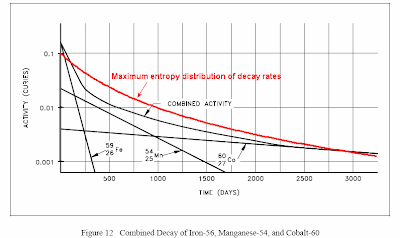[[ Check out my Wordpress blog Context/Earth for environmental and energy topics tied together in a semantic web framework ]]

## Friday, May 21, 2010

### Waste Half-Life

The big Gulf Spill got me thinking about the half-life of the leaking crude oil and the expanding slick. First of all, the oil will biodegrade over time. We don't have the situation as in CO2 where a sizable fraction will wander around the atmosphere trying to find a suitable location to react and form solutes.

Most of the oil will stay on the surface where it will get plenty of attention from aerobic microoganisms. Some of the oil will sink into the ocean and find anaerobic conditions at the bottom and essentially become inert or wash up on shore as sticky globs. Also the composition of crude oil includes many different hydrocarbons, some of which biodegrade at much slower rates, due to their molecular structure.

So I imagine that we can't calculate the half-life of the spilled oil in terms of a single rate constant, k. This kind of first-order kinetics would likely show an exponential decline, which proceeds pretty quickly once you get past the half-lifetime, 1/k . Instead we will get a mix of various rates, with the fast rates occurring initially and the slower rates picking up the slack.

Radioactive waste-dumps also show a mix of decay constants. Nominally, radioactive material will show a single Poisson emission rate, leading to an exponential decline over time. But when the different radioactive materials get combined, the Geiger counter will pick up this mixture of rates, and the decline will turn from an exponential to a fat tail distribution See the red curve below.A maximum entropy mix of decay rates (where a high decay rate indicates a potentially more energetic state) will generate the following half-life decline profile:
P(t) = 1/(1+k*t)
where k is the average of the individual rates. This looks exactly the same as the hyperbolic decline of reservoirs in my last post.

As you can see, the combined activity shows a much larger equivalent half-life since the tail has so much meat in it. In the limit of a full dispersion of rate constants, the average half-life will actually slowly diverge as the log of infinity. However, it never reaches this because the slowest decay rate will eventually dominate and that will not diverge.

In any case, this gives a good qualitative description of a random waste dump.

If I make the same MaxEnt assumption for crude oil and assume that the most energetic oil (by the bond strength of the hydrocarbon ) will likely prove the most difficult to decompose, then the half-life may also show a similar kind of fat-tail as that of a waste dump. It looks like benzene breaks down much slower than diesel oil for example.

As usual, disordered natural phenomena show many of the same dispersive characteristics, driven largely by maximizing entropy.

Notes:

 For the derivation, we assume that we have a mean energy E0 and then a probability density function will show many small energies and progressively fewer high energies.
p(E) = exp(-E/Eo)/E0
but the decomposition rate R depends on E, so that
P(t) = integral of P(t|E)p(E) over all E
P(t|E) = exp(-kE*t)

P(t) = 1/(1+tkEo)

(See this for a more detailed derivation.)